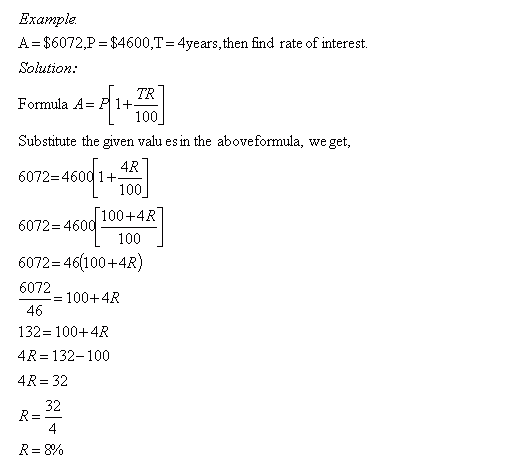Email us to get an instant 20% discount on highly effective K-12 Math & English kwizNET Programs!

Online Quiz (WorksheetABCD)

Questions Per Quiz = 2 4 6 8 10

Grade 8 - Mathematics9.32 Simple Interest - VIIIDirections: Solve the following problems. Also write at least ten examples of your own.
 Q 1: A = \$5304, P = \$4800 and T = 3years, then find rate of interest.3.5%2.5%2%4.5% Q 2: A = \$8284, P = \$7600 and T = 3years, then find rate of interest.3%3.5%2.5%4% Q 3: A = \$16352, P = \$14600 and T = 6years, then find rate of interest.1.5%3%2%4.5% Q 4: A = \$5428, P = \$4600 and T = 2years, then find rate of interest.9%6%8%5% Question 5: This question is available to subscribers only! Question 6: This question is available to subscribers only!